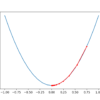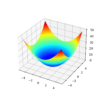# Archive | Optimization## A Gentle Introduction to Stochastic Optimization Algorithms

Stochastic optimization refers to the use of randomness in the objective function or in the optimization algorithm. Challenging optimization algorithms, such as high-dimensional nonlinear objective problems, may contain multiple local optima in which deterministic optimization algorithms may get stuck. Stochastic optimization algorithms provide an alternative approach that permits less optimal local decisions to be made […]## How to Use Optimization Algorithms to Manually Fit Regression Models

Regression models are fit on training data using linear regression and local search optimization algorithms. Models like linear regression and logistic regression are trained by least squares optimization, and this is the most efficient approach to finding coefficients that minimize error for these models. Nevertheless, it is possible to use alternate optimization algorithms to fit […]## Function Optimization With SciPy

Optimization involves finding the inputs to an objective function that result in the minimum or maximum output of the function. The open-source Python library for scientific computing called SciPy provides a suite of optimization algorithms. Many of the algorithms are used as a building block in other algorithms, most notably machine learning algorithms in the […]## Gradient Descent With Momentum from Scratch

Gradient descent is an optimization algorithm that follows the negative gradient of an objective function in order to locate the minimum of the function. A problem with gradient descent is that it can bounce around the search space on optimization problems that have large amounts of curvature or noisy gradients, and it can get stuck […]## Local Optimization Versus Global Optimization

Optimization refers to finding the set of inputs to an objective function that results in the maximum or minimum output from the objective function. It is common to describe optimization problems in terms of local vs. global optimization. Similarly, it is also common to describe optimization algorithms or search algorithms in terms of local vs. […]## How to Use Nelder-Mead Optimization in Python

The Nelder-Mead optimization algorithm is a widely used approach for non-differentiable objective functions. As such, it is generally referred to as a pattern search algorithm and is used as a local or global search procedure, challenging nonlinear and potentially noisy and multimodal function optimization problems. In this tutorial, you will discover the Nelder-Mead optimization algorithm. […]## Visualization for Function Optimization in Python

Function optimization involves finding the input that results in the optimal value from an objective function. Optimization algorithms navigate the search space of input variables in order to locate the optima, and both the shape of the objective function and behavior of the algorithm in the search space are opaque on real-world problems. As such, […]## Code Adam Optimization Algorithm From Scratch

Gradient descent is an optimization algorithm that follows the negative gradient of an objective function in order to locate the minimum of the function. A limitation of gradient descent is that a single step size (learning rate) is used for all input variables. Extensions to gradient descent like AdaGrad and RMSProp update the algorithm to […]## 3 Books on Optimization for Machine Learning

Optimization is a field of mathematics concerned with finding a good or best solution among many candidates. It is an important foundational topic required in machine learning as most machine learning algorithms are fit on historical data using an optimization algorithm. Additionally, broader problems, such as model selection and hyperparameter tuning, can also be framed […]## Univariate Function Optimization in Python

How to Optimize a Function with One Variable? Univariate function optimization involves finding the input to a function that results in the optimal output from an objective function. This is a common procedure in machine learning when fitting a model with one parameter or tuning a model that has a single hyperparameter. An efficient algorithm […]